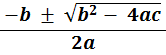### Solving quadratics of the form ax2 + bx + c = 0 − using the quadratic formula

Solving quadratics of the form ax2 + bx + c = 0 using the quadratic formula:

 x =In the examples that follow a is NOT equal to 1

• #### Example 1.   Solve 2x2 + 12x + 10 = 0

•  x =Verify by putting these two values of x into 2x2 + 12x + 10

• #### Example 2.   Solve 4x2 − 11x + 6 = 0

•  x =Check by putting these two values of x into 4x2 − 11x + 6

• #### Example 3.   Solve 2x2 + 2x − 24 = 0

•  x =Verify by putting these two values of x into 2x2 + 2x − 24

• #### Example 4.   Solve 3x2 − 4x − 20 = 0

•  x =Check by putting these two values of x into 3x2 − 4x − 20

#### Remember:Find the values of x where ax2 + bx + c = 0 is the same as solve ax2 + bx + c = 0to: